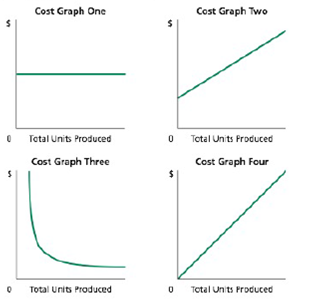# Identify cost graphs The following cost graphs illustrate various types of cost behavior: a. Total direct materials cost b. Electricity costs of \$1,000 per month plus 50.10 per kilowatt-hour c. Per-unit cost of straight-line depreciation on factory equipment d. Salary of quality control supervisor, 520,000 per month e. Per-unit direct labor cost### Accounting

27th Edition
WARREN + 5 others
Publisher: Cengage Learning,
ISBN: 9781337272094### Accounting

27th Edition
WARREN + 5 others
Publisher: Cengage Learning,
ISBN: 9781337272094

#### Solutions

Chapter
Section
Chapter 21, Problem 21.2EX
Textbook Problem

## Identify cost graphsThe following cost graphs illustrate various types of cost behavior:a. Total direct materials costb. Electricity costs of \$1,000 per month plus 50.10 per kilowatt-hourc. Per-unit cost of straight-line depreciation on factory equipmentd. Salary of quality control supervisor, 520,000 per monthe. Per-unit direct labor cost

Expert Solution
To determine

Cost Behavior: It refers to the method in which a cost changes with respect to the changes in its related activities. This method helps the management in estimating costs for decision making and predict future profits.

To identify: the cost graph for each of the given costs that best illustrates its cost behavior as the number of units produced increases.

### Explanation of Solution

Identify the cost graph for each of the given costs that best illustrates its cost behavior as the number of units produced increases.

a.

For total direct materials cost, the cost graph is four. It is a variable cost. The variable cost that starts at the point \$0 on the vertical axis (total cost) increases in an upward direction with the increase in the number of units produced shown on the horizontal axis.

b.

For electricity costs of \$1,000 per month plus \$0.10 per kilowatt-hour, the cost graph is two. It is a mixed cost that has both the features of fixed and variable cost. The total cost starts from the fixed cost of \$10,000, increasing in an upward direction at the rate of \$0.10 per-kilowatt-hour on the vertical axis to the maximum relevant range of the units produced per hour on the horizontal axis.

c.

For per-unit cost of straight-line depreciation on factory equipment, the cost graph is three...

### Want to see the full answer?

Check out a sample textbook solution.See solution

### Want to see this answer and more?

Bartleby provides explanations to thousands of textbook problems written by our experts, many with advanced degrees!

See solution